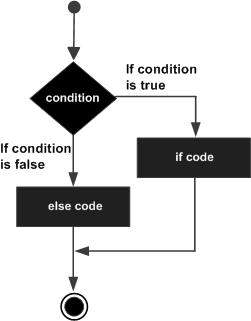# R - If...Else Statement

#### R Programming Online Training

40 Lectures 1.5 hours

#### Support Vector Machines in R (SVM in R)

27 Lectures 3 hours

#### Data Visualization using R Programming

14 Lectures 10 hours

An if statement can be followed by an optional else statement which executes when the boolean expression is false.

## Syntax

The basic syntax for creating an if...else statement in R is −

```if(boolean_expression) {
// statement(s) will execute if the boolean expression is true.
} else {
// statement(s) will execute if the boolean expression is false.
}
```

If the Boolean expression evaluates to be true, then the if block of code will be executed, otherwise else block of code will be executed.

## Flow Diagram## Example

```x <- c("what","is","truth")

if("Truth" %in% x) {
print("Truth is found")
} else {
}
```

When the above code is compiled and executed, it produces the following result −

``` "Truth is not found"
```

Here "Truth" and "truth" are two different strings.

## The if...else if...else Statement

An if statement can be followed by an optional else if...else statement, which is very useful to test various conditions using single if...else if statement.

When using if, else if, else statements there are few points to keep in mind.

• An if can have zero or one else and it must come after any else if's.

• An if can have zero to many else if's and they must come before the else.

• Once an else if succeeds, none of the remaining else if's or else's will be tested.

## Syntax

The basic syntax for creating an if...else if...else statement in R is −

```if(boolean_expression 1) {
// Executes when the boolean expression 1 is true.
} else if( boolean_expression 2) {
// Executes when the boolean expression 2 is true.
} else if( boolean_expression 3) {
// Executes when the boolean expression 3 is true.
} else {
// executes when none of the above condition is true.
}
```

## Example

```x <- c("what","is","truth")

if("Truth" %in% x) {
print("Truth is found the first time")
} else if ("truth" %in% x) {
print("truth is found the second time")
} else {
print("No truth found")
}
```

When the above code is compiled and executed, it produces the following result −

``` "truth is found the second time"
```
r_decision_making.htm